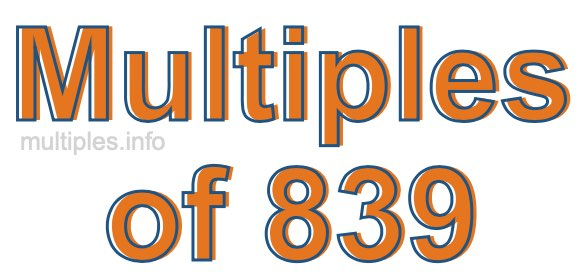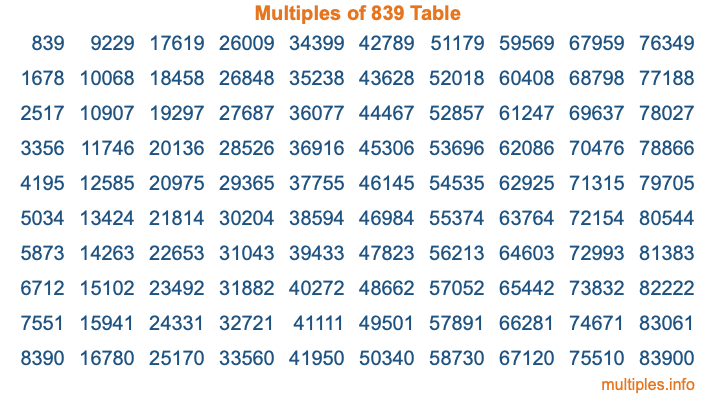Multiples of 839Welcome to the Multiples of 839 page. Here we will first teach you everything you will ever need to know about the multiples of 839, and then give you a study guide summary of everything we taught you to make sure you remember it all. Use this page to look up facts and learn information about the multiples of 839. This page will make you a multiples of eight hundred thirty-nine expert!

Definition of Multiples of 839
Multiples of 839 are all the numbers that when divided by 839 equal an integer. Each of the multiples of 839 are called a multiple. A multiple of 839 is created by multiplying 839 by an integer.

Therefore, to create a list of multiples of 839, you start with 1 multiplied by 839, then 2 multiplied by 839, then 3 multiplied by 839, and so on for as long as you want. Thus, the list of the first five multiples of 839 is 839, 1678, 2517, 3356, and 4195. To see a larger list of multiples of 839, see the printable image of Multiples of 839 further down on this page. We also have a category where you can choose any nth multiple of 839.

Multiples of 839 Checker
The Multiples of 839 Checker below checks to see if any number of your choice is a multiple of 839. In other words, it checks to see if there is any number (integer) that when multiplied by 839 will equal your number. To do that, we divide your number by 839. If the the quotient is an integer, then your number is a multiple of 839.

Is  a multiple of 839?

Least Common Multiple of 839 and ...
A Least Common Multiple (LCM) is the lowest multiple that two or more numbers have in common. This is also called the smallest common multiple or lowest common multiple and is useful to know when you are adding our subtracting fractions. Enter one or more numbers below (839 is already entered) to find the LCM.

Check out our LCM Calculator if you need more details about the Least Common Multiple or if you need the LCM for different numbers for adding and subtraction fractions.

nth Multiple of 839
As we stated above, 839 is the first multiple of 839, 1678 is the second multiple of 839, 2517 is the third multiple of 839, and so on. Enter a number below to find the nth multiple of 839.

th multiple of 839

Multiples of 839 vs Factors of 839
839 is a multiple of 839 and a factor of 839, but that is where the similarities end. All postive multiples of 839 are 839 or greater than 839. All positive factors of 839 are 839 or less than 839.

Below is the beginning list of multiples of 839 and the factors of 839 so you can compare:

Multiples of 839: 839, 1678, 2517, 3356, 4195, etc.

Factors of 839: 1, 839

As you can see, the multiples of 839 are all the numbers that you can divide by 839 to get a whole number. The factors of 839, on the other hand, are all the whole numbers that you can multiply by another whole number to get 839.

It's also interesting to note that if a number (x) is a factor of 839, then 839 will also be a multiple of that number (x).

Multiples of 839 vs Divisors of 839
The divisors of 839 are all the integers that 839 can be divided by evenly. Below is a list of the divisors of 839.

Divisors of 839: 1, 839

The interesting thing to note here is that if you take any multiple of 839 and divide it by a divisor of 839, you will see that the quotient is an integer.

Multiples of 839 Table
Below is an image of the first 100 multiples of 839 in a table. The table is in chronological order, column by column. The first column has the first ten multiples of 839, the second column has the next ten multiples of 839, and so on.The Multiples of 839 Table is also referred to as the 839 Times Table or Times Table of 839. You are welcome to print out our table for your studies.

Negative Multiples of 839
Although not often discussed or needed in math, it is worth mentioning that you can make a list of negative multiples of 839 by multiplying 839 by -1, then by -2, then by -3, and so on, to get the following list of negative multiples of 839:

-839, -1678, -2517, -3356, -4195, etc.

Multiples of 839 Summary
Below is a summary of important Multiples of 839 facts that we have discussed on this page. To retain the knowledge on this page, we recommend that you read through the summary and explain to yourself or a study partner why they hold true.

There are an infinite number of multiples of 839.

A multiple of 839 divided by 839 will equal a whole number.

839 divided by a factor of 839 equals a divisor of 839.

The nth multiple of 839 is n times 839.

The largest factor of 839 is equal to the first positive multiple of 839.

839 is a multiple of every factor of 839.

839 is a multiple of 839.

A multiple of 839 divided by a divisor of 839 equals an integer.

839 divided by a divisor of 839 equals a factor of 839.

Any integer times 839 will equal a multiple of 839.

Multiples of a Number
Here you can get the multiples of another number, all with the same attention to detail as we did for multiples of 839 on this page.

Multiples of
Multiples of 840
Did you find our page about multiples of eight hundred thirty-nine educational? Do you want more knowledge? Check out the multiples of the next number on our list!